# The Universe of Discourse

Tue, 31 Oct 2017

[ The Atom and RSS feeds have done an unusually poor job of preserving the mathematical symbols in this article. It will be much more legible if you read it on my blog. ]

Lately I've been enjoying The Blind Spot by Jean-Yves Girard, a very famous logician. (It is translated from French; the original title is Le Point Aveugle.) This is an unusual book. It is solidly full of deep thought and technical detail about logic, but it is also opinionated, idiosyncratic and polemical. Chapter 2 (“Incompleteness”) begins:

It is out of question to enter into the technical arcana of Gödel’s theorem, this for several reasons:

(i) This result, indeed very easy, can be perceived, like the late paintings of Claude Monet, but from a certain distance. A close look only reveals fastidious details that one perhaps does not want to know.

(ii) There is no need either, since this theorem is a scientific cul-de-sac : in fact it exposes a way without exit. Since it is without exit, nothing to seek there, and it is of no use to be expert in Gödel’s theorem.

(The Blind Spot, p. 29)

He continues a little later:

Rather than insisting on those tedious details which «hide the forest», we shall spend time on objections, from the most ridiculous to the less stupid (none of them being eventually respectable).

As you can see, it is not written in the usual dry mathematical-text style, presenting the material as a perfect and aseptic distillation of absolute truth. Instead, one sees the history of logic, the rise and fall of different theories over time, the interaction and relation of many mathematical and philosophical ideas, and Girard's reflections about it all. It is a transcription of a lecture series, and reads like one, including all of the speaker's incidental remarks and offhand musings, but written down so that each can be weighed and pondered at length. Instead of wondering in the moment what he meant by some intriguing remark, then having to abandon the thought to keep up with the lecture, I can pause and ponder the significance. Girard is really, really smart, and knows way more about logic than I ever will, and his offhand remarks reward this pondering. The book is profound in a way that mathematics books often aren't. I wanted to provide an illustrative quotation, but to briefly excerpt a profound thought is to destroy its profundity, so I will have to refrain.)

The book really gets going with its discussion of Gentzen's sequent calculus in chapter 3.

Between around 1890 (when Peano and Frege began to liberate logic from its medieval encrustations) and 1935 when the sequent calculus was invented, logical proofs were mainly in the “Hilbert style”. Typically there were some axioms, and some rules of deduction by which the axioms could be transformed into other formulas. A typical example consists of the axioms $$A\to(B\to A)\\ (A \to (B \to C)) \to ((A \to B) \to (A \to C))$$ (where !!A, B, C!! are understood to be placeholders that can be replaced by any well-formed formulas) and the deduction rule modus ponens: having proved !!A\to B!! and !!A!!, we can deduce !!B!!.

In contrast, sequent calculus has few axioms and many deduction rules. It deals with sequents which are claims of implication. For example: $$p, q \vdash r, s$$ means that if we can prove all of the formulas on the left of the ⊢ sign, then we can conclude some of the formulas on the right. (Perhaps only one, but at least one.)

A typical deductive rule in sequent calculus is:

$$\begin{array}{c} Γ ⊢ A, Δ \qquad Γ ⊢ B, Δ \\ \hline Γ ⊢ A ∧ B, Δ \end{array}$$

Here !!Γ!! and !!Δ!! represent any lists of formulas, possibly empty. The premises of the rule are:

1. !!Γ ⊢ A, Δ!!: If we can prove all of the formulas in !!Γ!!, then we can conclude either the formula !!A!! or some of the formulas in !!Δ!!.

2. !!Γ ⊢ B, Δ!!: If we can prove all of the formulas in !!Γ!!, then we can conclude either the formula !!B!! or some of the formulas in !!Δ!!.

From these premises, the rule allows us to deduce:

!!Γ ⊢ A ∧ B, Δ!!: If we can prove all of the formulas in !!Γ!!, then we can conclude either the formula !!A \land B!! or some of the formulas in !!Δ!!.

The only axioms of sequent calculus are utterly trivial:

$$\begin{array}{c} \phantom{A} \\ \hline A ⊢ A \end{array}$$

There are no premises; we get this deduction for free: If can prove !!A!!, we can prove !!A!!. (!!A!! here is a metavariable that can be replaced with any well-formed formula.)

One important point that Girard brings up, which I had never realized despite long familiarity with sequent calculus, is the symmetry between the left and right sides of the turnstile ⊢. As I mentioned, the interpretation of !!Γ ⊢ Δ!! I had been taught was that it means that if every formula in !!Γ!! is provable, then some formula in !!Δ!! is provable. But instead let's focus on just one of the formulas !!A!! on the right-hand side, hiding in the list !!Δ!!. The sequent !!Γ ⊢ Δ, A!! can be understood to mean that to prove !!A!!, it suffices to prove all of the formulas in !!Γ!!, and to disprove all the formulas in !!Δ!!. And now let's focus on just one of the formulas on the left side: !!Γ, A ⊢ Δ!! says that to disprove !!A!!, it suffices to prove all the formulas in !!Γ!! and disprove all the formulas in !!Δ!!.

The all-some correspondence, which had previously caused me to wonder why it was that way and not something else, perhaps the other way around, has turned into a simple relationship about logical negation: the formulas on the left are positive, and the ones on the right are negative.) With this insight, the sequent calculus negation laws become not merely simple but trivial:

$$\begin{array}{cc} \begin{array}{c} Γ, A ⊢ Δ \\ \hline Γ ⊢ \lnot A, Δ \end{array} & \qquad \begin{array}{c} Γ ⊢ A, Δ \\ \hline Γ, \lnot A ⊢ Δ \end{array} \end{array}$$

For example, in the right-hand deduction: what is sufficient to prove !!A!! is also sufficient to disprove !!¬A!!.

(Compare also the rule I showed above for ∧: It now says that if proving everything in !!Γ!! and disproving everything in !!Δ!! is sufficient for proving !!A!!, and likewise sufficient for proving !!B!!, then it is also sufficient for proving !!A\land B!!.)

I never really appreciated the cut rule before. Most of the deductive rules in the sequent calculus are intuitively plausible and so simple and obvious that it is easy to imagine coming up with them oneself.

But the cut rule is more complicated than the rules I have already shown. I don't think I would have thought of it easily:

$$\begin{array}{c} Γ ⊢ A, Δ \qquad Λ, A ⊢ Π \\ \hline Γ, Λ ⊢ Δ, Π \end{array}$$

(Here !!A!! is a formula and !!Γ, Δ, Λ, Π!! are lists of formulas, possibly empty lists.)

Girard points out that the cut rule is a generalization of modus ponens: taking !!Γ, Δ, Λ!! to be empty and !!Π = \{B\}!! we obtain:

$$\begin{array}{c} ⊢ A \qquad A ⊢ B \\ \hline ⊢ B \end{array}$$

The cut rule is also a generalization of the transitivity of implication:

$$\begin{array}{c} X ⊢ A \qquad A ⊢ Y \\ \hline X ⊢ Y \end{array}$$

Here we took !!Γ = \{X\}, Π = \{Y\}!!, and !!Δ!! and !!Λ!! empty.

This all has given me a much better idea of where the cut rule came from and why we have it.

In sequent calculus, the deduction rules all come in pairs. There is a rule about introducing ∧, which I showed before. It allows us to construct a sequent involving a formula with an ∧, where perhaps we had no ∧ before. (In fact, it is the only way to do this.) There is a corresponding rule (actually two rules) for getting rid of ∧ when we have it and we don't want it:

$$\begin{array}{cc} \begin{array}{c} Γ ⊢ A\land B, Δ \\ \hline Γ ⊢ A, Δ \end{array} & \qquad \begin{array}{c} Γ ⊢ A\land B, Δ \\ \hline Γ ⊢ B, Δ \end{array} \end{array}$$

Similarly there is a rule (actually two rules) about introducing !!\lor!! and a corresponding rule about eliminating it.

The cut rule seems to lie outside this classification. It is not paired.

But Girard showed me that it is part of a pair. The axiom

$$\begin{array}{c} \phantom{A} \\ \hline A ⊢ A \end{array}$$

can be seen as an introduction rule for a pair of !!A!!s, one on each side of the turnstile. The cut rule is the corresponding rule for eliminating !!A!! from both sides.

Sequent calculus proofs are much easier to construct than Hilbert-style proofs. Suppose one wants to prove !!B!!. In a Hilbert system the only deduction rule is modus ponens, which requires that we first prove !!A\to B!! and !!A!! for some !!A!!. But what !!A!! should we choose? It could be anything, and we have no idea where to start or how big it could be. (If you enjoy suffering, try to prove the simple theorem !!A\to A!! in the Hilbert system I described at the beginning of the article. (Solution)

In sequent calculus, there is only one way to prove each kind of thing, and the premises in each rule are simply related to the consequent we want. Constructing the proof is mostly a matter of pushing the symbols around by following the rules to their conclusions. (Or, if this is impossible, one can conclude that there is no proof, and why.) Construction of proofs can now be done entirely mechanically!

Except! The cut rule does require one to guess a formula: If one wants to prove !!Γ, Λ ⊢ Δ, Π!!, one must guess what !!A!! should appear in the premises !!Γ, A ⊢ Δ!! and !!Λ ⊢ A, Π!!. And there is no constraint at all on !!A!!; it could be anything, and we have no idea where to start or how big it could be.

The good news is that Gentzen, the inventor of sequent calculus, showed that one can dispense with the cut rule: it is unnecessary:

In Hilbert-style systems, based on common sense, the only rule is (more or less) the Modus Ponens : one reasons by linking together lemmas and consequences. We just say that one can get rid of that : it is like crossing the English Channel with fists and feet bound !

(The Blind Spot, p. 61)

Gentzen's demonstration of this shows how one can take any proof that involves the cut rule, and algorithmically eliminate the cut rule from it to obtain a proof of the same result that does not use cut. Gentzen called this the “Hauptsatz” (“principal theorem”) and rightly so, because it reduces construction of logical proofs to an algorithm and is therefore the ultimate basis for algorithmic proof theory.

The bad news is that the cut-elimination process can super-exponentially increase the size of the proof, so it does not lead to a practical algorithm for deciding provability. Girard analyzed why, and what he discovered amazed me. The only problem is in the contraction rules, which had seemed so trivial and innocuous—uninteresting, even—that I had never given them any thought:

$$\begin{array}{cc} \begin{array}{c} Γ, A, A ⊢ Δ \\ \hline Γ, A ⊢ Δ \end{array} & \qquad \begin{array}{c} Γ ⊢ A, A, Δ \\ \hline Γ ⊢ A, Δ \end{array} \end{array}$$

And suddenly Girard's invention of linear logic made sense to me. In linear logic, contraction is forbidden; one must use each formula in one and only one deduction.

Previously it had seemed to me that this was a pointless restriction. Now I realized that it was no more of a useless hair shirt than the intuitionistic rejection of the law of the proof by contradiction: not a stubborn refusal to use an obvious tool of reasoning, but a restriction of proofs to produce better reasoning. With the rejection of contraction, cut-elimination no longer explodes proof size, and automated theorem proving becomes practical:

This is why its study is, implicitly, at the very heart of these lectures.

(The Blind Spot, p. 63)

The book is going to get into linear logic later in the next chapter. I have read descriptions of linear logic before, but never understood what it was up to. (It has two logical and operators, and two logical or operators; why?) But I am sure Girard will explain it marvelously.

1. In place of a profound excerpt, I will present the following, which isn't especially profound but struck a chord for me: “By the way, nothing is more arbitrary than a modal logic « I am done with this logic, may I have another one ? » seems to be the motto of these guys.” (p. 24)
2. Compare my alternative representation of arithmetic expressions that collapses addition and subtraction, and multiplication and division.
3. A typically Girardian remark is that analytic tableaux are “the poor man's sequents”.

Sun, 15 Oct 2017

Back in February I posted an article about how there are exactly 715 nondecreasing sequences of 4 digits. I said that !!S(10, 4)!! was the set of such sequences and !!C(10, 4)!! was the number of such sequences, and in general $$C(d,n) = \binom{n+d-1}{d-1} = \binom{n+d-1}{n}$$ so in particular $$C(10,4) = \binom{13}{4} = 715.$$

I described more than one method of seeing this, but I didn't mention the method I had found first, which was to use the Cauchy-Frobenius-Redfeld-Pólya-Burnside counting lemma. I explained the lemma in detail some time ago, with beautiful illustrated examples, so I won't repeat the explanation here. The Burnside lemma is a kind of big hammer to use here, but I like big hammers. And the results of this application of the big hammer are pretty good, and justify it in the end.

To count the number of distinct sequences of 4 digits, where some sequences are considered “the same” we first identify a symmetry group whose orbits are the equivalence classes of sequences. Here the symmetry group is !!S_4!!, the group that permutes the elements of the sequence, because two sequences are considered “the same” if they have exactly the same digits but possibly in a different order, and the elements of !!S_4!! acting on the sequences are exactly what you want to permute the elements into some different order.

Then you tabulate how many of the 10,000 original sequences are left fixed by each element !!p!! of !!S_4!!, which is exactly the number of cycles of !!p!!. (I have also discussed cycle classes of permutations before.) If !!p!! contains !!n!! cycles, then !!p!! leaves exactly !!10^n!! of the !!10^4!! sequences fixed.

 Cycle class Numberof cycles How manypermutations? Sequencesleft fixed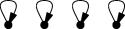4 1 10,000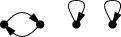3 6 1,000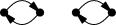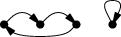2 3 + 8 = 11 100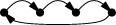1 6 10 24 17,160

(Skip this paragraph if you already understand the table. The four rows above are an abbreviation of the full table, which has 24 rows, one for each of the 24 permutations of order 4. The “How many permutations?” column says how many times each row should be repeated. So for example the second row abbreviates 6 rows, one for each of the 6 permutations with three cycles, which each leave 1,000 sequences fixed, for a total of 6,000 in the second row, and the total for all 24 rows is 17,160. There are two different types of permutations that have two cycles, with 3 and 8 permutations respectively, and I have collapsed these into a single row.)

Then the magic happens: We average the number left fixed by each permutation and get !!\frac{17160}{24} = 715!! which we already know is the right answer.

Now suppose we knew how many permutations there were with each number of cycles. Let's write !!\def\st#1#2{\left[{#1\atop #2}\right]}\st nk!! for the number of permutations of !!n!! things that have exactly !!k!! cycles. For example, from the table above we see that $$\st 4 4 = 1,\quad \st 4 3 = 6,\quad \st 4 2 = 11,\quad \st 4 1 = 6.$$

Then applying Burnside's lemma we can conclude that $$C(d, n) = \frac1{n!}\sum_i \st ni d^i .\tag{\spadesuit}$$ So for example the table above computes !!C(10,4) = \frac1{24}\sum_i \st 4i 10^i = 715!!.

At some point in looking into this I noticed that $$\def\rp#1#2{#1^{\overline{#2}}}% \def\fp#1#2{#1^{\underline{#2}}}% C(d,n) = \frac1{n!}\rp dn$$ where !!\rp dn!! is the so-called “rising power” of !!d!!: $$\rp dn = d\cdot(d+1)(d+2)\cdots(d+n-1).$$ I don't think I had a proof of this; I just noticed that !!C(d, 1) = d!! and !!C(d, 2) = \frac12(d^2+d)!! (both obvious), and the Burnside's lemma analysis of the !!n=4!! case had just given me !!C(d, 4) = \frac1{24}(d^4 +6d^3 + 11d^2 + 6d)!!. Even if one doesn't immediately recognize this latter polynomial it looks like it ought to factor and then on factoring it one gets !!d(d+1)(d+2)(d+3)!!. So it's easy to conjecture !!C(d, n) = \frac1{n!}\rp dn!! and indeed, this is easy to prove from !!(\spadesuit)!!: The !!\st n k!! obey the recurrence $$\st{n+1}k = n \st nk + \st n{k-1}\tag{\color{green}{\star}}$$ (by an easy combinatorial argument1) and it's also easy to show that the coefficients of !!\rp nk!! obey the same recurrence.2

In general !!\rp nk = \fp{(n+k-1)}k!! so we have !!C(d, n) = \rp dn = \fp{(n+d-1)}n = \binom{n+d-1}d = \binom{n+d-1}{n-1}!! which ties the knot with the formula from the previous article. In particular, !!C(10,4) = \binom{13}9!!.

 We want to show that the coefficients of !!\rp nk!! obey the same recurrence as !!(\color{green}{\star})!!. Let's say that the coefficient of the !!n^i!! term in !!\rp nk!! is !!c_i!!. We have $$\rp n{k+1} = \rp nk\cdot (n+k) = \rp nk \cdot n + \rp nk \cdot k$$ so the coefficient of the the !!n^i!! term on the left is !!c_{i-1} + kc_i!!.